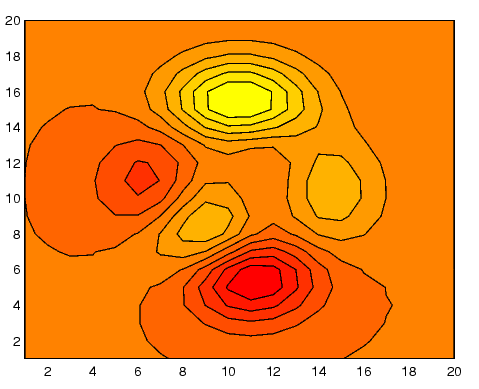MATLAB Function Referencecontourf

Filled two-dimensional contour plot

Syntax

• ```contourf(Z)
contourf(Z,n)
contourf(Z,v)
contourf(X,Y,Z)
contourf(X,Y,Z,n)
contourf(X,Y,Z,v)
contourf(axes_handle,...)
[C,h,CF] = contourf(...)
```

Description

A filled contour plot displays isolines calculated from matrix `Z` and fills the areas between the isolines using constant colors. The color of the filled areas depends on the current figure's colormap.

```contourf(Z) ``` draws a contour plot of matrix `Z`, where `Z` is interpreted as heights with respect to a plane. `Z` must be at least a 2-by-2 matrix. The number of contour lines and the values of the contour lines are chosen automatically.

```contourf(Z,n) ``` draws a contour plot of matrix `Z` with `n` contour levels.

```contourf(Z,v) ``` draws a contour plot of matrix `Z` with contour levels at the values specified in vector `v`.

```contourf(X,Y,Z), contourf(X,Y,Z,n), and contourf(X,Y,Z,v) ``` produce contour plots of `Z` using `X` and `Y` to determine the x- and y-axis limits. When `X` and `Y` are matrices, they must be the same size as `Z`, in which case they specify a surface as `surf` does.

```contourf(axes_handle,...) ``` plots into the axes with handle `axes_handle` instead of the current axes (`gca`).

```[C,h,CF] = contourf(...) ``` returns the contour matrix `C` as calculated by the function `contourc` and used by `clabel`, a vector of handles `h` to patch graphics objects, and a contour matrix `CF` for the filled areas.

Remarks

If `X` or `Y` is irregularly spaced, `contourf` calculates contours using a regularly spaced contour grid, then transforms the data to `X` or `Y`.

Examples

Create a filled contour plot of the `peaks` function.

• ```[C,h] = contourf(peaks(20),10);
colormap autumn```

See Also

`clabel`, `contour`, `contour3`, `contourc`, `quiver`

Contour Plots for related functionscontourc Contourgroup Properties© 1994-2005 The MathWorks, Inc.